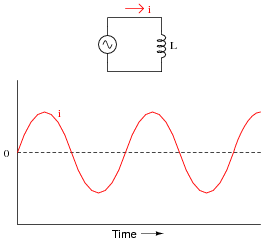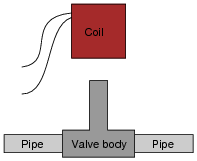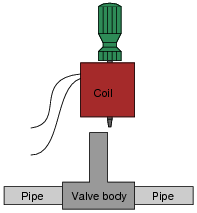# Inductive Reactance

## AC Electric Circuits

• #### Question 1

 Don’t just sit there! Build something!!

Learning to mathematically analyze circuits requires much study and practice. Typically, students practice by working through lots of sample problems and checking their answers against those provided by the textbook or the instructor. While this is good, there is a much better way.

You will learn much more by actually building and analyzing real circuits, letting your test equipment provide the “answers” instead of a book or another person. For successful circuit-building exercises, follow these steps:

1. Carefully measure and record all component values prior to circuit construction.
2. Draw the schematic diagram for the circuit to be analyzed.
3. Carefully build this circuit on a breadboard or other convenient medium.
4. Check the accuracy of the circuit’s construction, following each wire to each connection point, and verifying these elements one-by-one on the diagram.
5. Mathematically analyze the circuit, solving for all voltage and current values.
6. Carefully measure all voltages and currents, to verify the accuracy of your analysis.
7. If there are any substantial errors (greater than a few percent), carefully check your circuit’s construction against the diagram, then carefully re-calculate the values and re-measure.

For AC circuits where inductive and capacitive reactances (impedances) are a significant element in the calculations, I recommend high quality (high-Q) inductors and capacitors, and powering your circuit with low frequency voltage (power-line frequency works well) to minimize parasitic effects. If you are on a restricted budget, I have found that inexpensive electronic musical keyboards serve well as “function generators” for producing a wide range of audio-frequency AC signals. Be sure to choose a keyboard “voice” that closely mimics a sine wave (the “panflute” voice is typically good), if sinusoidal waveforms are an important assumption in your calculations.

As usual, avoid very high and very low resistor values, to avoid measurement errors caused by meter “loading”. I recommend resistor values between 1 kΩ and 100 kΩ.

One way you can save time and reduce the possibility of error is to begin with a very simple circuit and incrementally add components to increase its complexity after each analysis, rather than building a whole new circuit for each practice problem. Another time-saving technique is to re-use the same components in a variety of different circuit configurations. This way, you won’t have to measure any component’s value more than once.

• #### Question 2

Suppose someone were to ask you to differentiate electrical reactance (X) from electrical resistance (R). How would you distinguish these two similar concepts from one another, using your own words?

• #### Question 3

As a general rule, inductors oppose change in (choose: voltage or current ), and they do so by . . . (complete the sentence).

Based on this rule, determine how an inductor would react to a constant AC current that increases in frequency. Would an inductor drop more or less voltage, given a greater frequency? Explain your answer.

• #### Question 4

We know that the formula relating instantaneous voltage and current in an inductor is this:

 e = L di dt

Knowing this, determine at what points on this sine wave plot for inductor current is the inductor voltage equal to zero, and where the voltage is at its positive and negative peaks. Then, connect these points to draw the waveform for inductor voltage:How much phase shift (in degrees) is there between the voltage and current waveforms? Which waveform is leading and which waveform is lagging?

• #### Question 5

Does an inductor’s opposition to alternating current increase or decrease as the frequency of that current increases? Also, explain why we refer to this opposition of AC current in an inductor as reactance instead of resistance.

• #### Question 6

What will happen to the brightness of the light bulb as the iron core is moved away from the wire coil in this circuit? Explain why this happens.• #### Question 7

An inductor rated at 4 Henrys is subjected to a sinusoidal AC voltage of 24 volts RMS, at a frequency of 60 hertz. Write the formula for calculating inductive reactance (XL), and solve for current through the inductor.

• #### Question 8

At what frequency does a 350 mH inductor have 4.7 kΩ of reactance? Write the formula for solving this, in addition to calculating the frequency.

• #### Question 9

How much inductance would an inductor have to possess in order to provide 540 Ω of reactance at a frequency of 400 Hz? Write the formula for solving this, in addition to calculating the frequency.

• #### Question 10

Explain all the steps necessary to calculate the amount of current in this inductive AC circuit:• #### Question 11

A solenoid valve is a mechanical shutoff device actuated by electricity. An electromagnet coil produces an attractive force on an iron “armature” which then either opens or closes a valve mechanism to control the flow of some fluid. Shown here are two different types of illustrations, both showing a solenoid valve:Some solenoid valves are constructed in such a way that the coil assembly may be removed from the valve body, separating these two pieces so that maintenance work may be done on one without interfering with the other. Of course, this means the valve mechanism will no longer be actuated by the magnetic field, but at least one piece may be worked upon without having to remove the other piece from whatever it may be connected to:This is commonly done when replacement of the valve mechanism is needed. First, the coil is lifted off the valve mechanism, then the maintenance technician is free to remove the valve body from the pipes and replace it with a new valve body. Lastly, the coil is re-installed on the new valve body and the solenoid is once more ready for service, all without having to electrically disconnect the coil from its power source.

However, if this is done while the coil is energized, it will overheat and burn up in just a few minutes. To prevent this from happening, the maintenance technicians have learned to insert a steel screwdriver through the center hole of the coil while it is removed from the valve body, like this:With the steel screwdriver shank taking the place of the iron armature inside the valve body, the coil will not overheat and burn up even if continually powered. Explain the nature of the problem (why the coil tends to burn up when separated from the valve body) and also why a screwdriver put in place of the iron armature works to prevent this from happening.

• #### Question 12

When AC power is initially applied to an electric motor (before the motor shaft has an opportunity to start moving), the motor “appears” to the AC power source to be a large inductor:If the voltage of the 60 Hz AC power source is 480 volts RMS, and the motor initially draws 75 amps RMS when the double-pole single-throw switch closes, how much inductance (L) must the motor windings have? Ignore any wire resistance, and assume the motor’s only opposition to current in a locked-rotor condition is inductive reactance (XL).

• #### Question 13

In analyzing circuits with inductors, we often take the luxury of assuming the constituent inductors to be perfect; i.e., purely inductive, with no “stray” properties such as winding resistance or inter-winding capacitance.

Real life is not so generous. With real inductors, we have to consider these factors. One measure often used to express the “purity” of an inductor is its so-called Q rating, or quality factor.

Write the formula for calculating the quality factor (Q) of a coil, and describe some of the operational parameters that may affect this number.